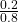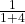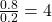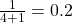Probability Concepts | IFT World
IFT Notes for Level I CFA® Program
IFT Notes for Level I CFA® Program

# Part 1

## 1. Probability Concepts and Odds Ratios

Since many investment decisions are made in an environment of uncertainty, it is essential for portfolio managers and investment managers to have a fundamental grasp of probability concepts. In this reading, we will focus on:

• Definitions and rules related to probability
• Expected value and variance
• Covariance and correlation

### Fundamental Concepts

A random variable is an uncertain quantity/number. For example, when you roll a die, the result is a random variable.

An outcome is the observed value of a random variable. For example, if you roll a 2, it is an outcome.

An event can be a single outcome or a set of outcomes. For example, you can define an event as rolling a 2 or rolling an even number.

Mutually exclusive events are events that cannot happen at the same time. For example, rolling a 2 and rolling a 3 are examples of mutually exclusive events. They cannot happen at the same time.

Exhaustive events are those that cover all possible outcomes. For example, ‘rolling an even number’ or’ rolling an odd number’ are exhaustive events. They cover all possible outcomes.

The two defining properties of probability are:

• The probability of any event has to be between 0 and 1.
• The sum of the probabilities of mutually exclusive and exhaustive events is equal to 1.

### Ways of Estimating Probability

The methods of estimating probabilities are:

• Empirical probability: Based on analyzing the frequency of an event’s occurrence in the past.
• A priori probability: Based on formal reasoning and inspection rather than personal judgment.
• Subjective probability: Informed guess based on personal judgment.

Empirical and a priori probabilities are often grouped as objective probabilities because they do not vary from person to person.

Probability Stated as Odds

Odds for an event are defined as the probability of the event occurring to the probability of the event not occurring. Odds for E = P(E) / [1 – P(E)].

Given odds for E of “a to b”, the implied probability of E is a / (a + b).

Example

If the probability of an event is 0.2, what are the odds of it occurring? Alternatively, if the odds are 1 to 4, what is the probability of this event?

Solution:

The odds of the event occurring are == 1/4. This is stated as odds of 1 to 4.

Given the odds, the probability of the event occurring is== 0.20.

Odds against an event are defined as the probability of the event not occurring to the probability of the event occurring. Odds against E = [1 – P(E)] / P(E).

Give odds against E of “a to b”, the implied probability of E is b / (a + b).

Example

If P(E) = 0.2, what are the odds against the event occurring? If the odds against an event are 4 to 1, what is the probability of the event?

Solution:

P(E) =, Hence the odds against E are 4 to 1.

Given the odds against an event, the probability of the event is## 2. Conditional and Joint Probability

### Conditional v/s Unconditional probabilities

Unconditional probability is the probability of an event occurring irrespective of the occurrence of other events. It is denoted as P(A). Unconditional probability is also called ‘marginal’ probability.

Conditional probability is the probability of an event occurring given that another event has occurred. It is denoted as P(A|B), which is the probability of event A given that event B has occurred.

### Joint Probability and Multiplication Rule

Multiplication rule is used to determine the joint probability of two events. It is expressed as:

P(AB) = P(A|B) P(B)

Rearranging the equation we get the formula for computing conditional probabilities:

P(A|B) = P(AB) / P(B)

Example

P(interest rates will decrease) = P(D) = 40%

P(stock price increases) = P(S)

P(stock price will increase given interest rates decrease) = P(S|D) = 70%

Compute probability of a stock price increase and an interest rate decrease.

Solution:

P(SD) = P(S|D) x P(D) = 0.7 x 0.4 = 0.28 = 28%

Addition rule is used to determine the probability that at least one of the events will occur. It is expressed as:

P(A or B) = P(A) + P(B) – P(AB)

P(AB) represents the joint probability that both A and B will occur. It is subtracted from the sum of the unconditional probabilities: P(A) + P(B), to avoid double counting.

If the two events are mutually exclusive, the joint probability: P(AB) is  zero and the probability that either A or B will occur is simply the sum of the unconditional probabilities for each event:

P(A or B) = P(A) + P(B)

Example

P(price of A increases) = P(A) = 0.5

P(price of B increases) = P(B) = 0.7

P(price of A and B increases) = P(AB) = 0.3

Compute the probability that the price of stock A or the price of stock B increases.

Solution

P(A or B) = 0.5 + 0.7 – 0.3 = 0.9